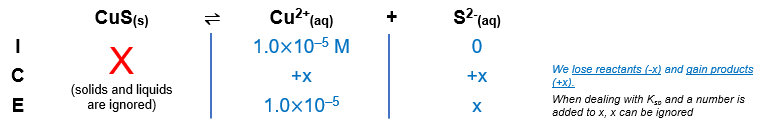# Problem: A solution is 0.010 M in both Cu2+ and Cd2+. What percentage of Cd2+ remains in the solution when 99.9% of the Cu2+ has been precipitated as CuS by adding sulfide?

###### FREE Expert Solution

Initial:

[Cu2+] = 0.010 M

[Cd2+] = 0.010 M

99.9% of Cu2+ has been precipitated as CuS

Cu2+ left = 100% - 99.9%
Cu2+ left = 0.1%

[Cu2+] = (0.010 M) (0.1%)
[Cu2+] = 1.0x10-5 M

Ksp CuS = 8.5x10-45   → CuS smaller Ksp → will precipitate first
Ksp CdS = 1.0x10-28

Calculate [S2-]:

CuS(s) → Cu2+(aq) + S2-(aq)${\mathbf{K}}_{\mathbf{sp}}\mathbf{=}\mathbf{\left[}{\mathbf{Cu}}^{\mathbf{2}\mathbf{+}}\mathbf{\right]}\mathbf{\left[}{\mathbf{S}}^{\mathbf{2}\mathbf{-}}\mathbf{\right]}\phantom{\rule{0ex}{0ex}}\mathbf{8}\mathbf{.}\mathbf{5}\mathbf{×}{\mathbf{10}}^{\mathbf{-}\mathbf{45}}\mathbf{=}\mathbf{\left(}\mathbf{1}\mathbf{.}\mathbf{0}\mathbf{×}{\mathbf{10}}^{\mathbf{-}\mathbf{5}}\mathbf{\right)}\mathbf{\left(}\mathbf{x}\mathbf{\right)}\phantom{\rule{0ex}{0ex}}\frac{\mathbf{8}\mathbf{.}\mathbf{5}\mathbf{×}{\mathbf{10}}^{\mathbf{-}\mathbf{45}}}{\mathbf{\left(}\mathbf{1}\mathbf{.}\mathbf{0}\mathbf{×}{\mathbf{10}}^{\mathbf{-}\mathbf{5}}\mathbf{\right)}}\mathbf{=}\frac{\overline{)\mathbf{\left(}\mathbf{1}\mathbf{.}\mathbf{0}\mathbf{×}{\mathbf{10}}^{\mathbf{-}\mathbf{5}}\mathbf{\right)}}\mathbf{\left(}\mathbf{x}\mathbf{\right)}}{\overline{)\mathbf{\left(}\mathbf{1}\mathbf{.}\mathbf{0}\mathbf{×}{\mathbf{10}}^{\mathbf{-}\mathbf{5}}\mathbf{\right)}}}$

x = 8.5x10-40

[S2-] = 8.5x10-40 M

Calculate minimum [S2-] to precipitate 0.010 M Cd2+:

97% (365 ratings)###### Problem Details

A solution is 0.010 M in both Cu2+ and Cd2+. What percentage of Cd2+ remains in the solution when 99.9% of the Cu2+ has been precipitated as CuS by adding sulfide?

Frequently Asked Questions

What scientific concept do you need to know in order to solve this problem?

Our tutors have indicated that to solve this problem you will need to apply the Selective Precipitation concept. If you need more Selective Precipitation practice, you can also practice Selective Precipitation practice problems.

What professor is this problem relevant for?

Based on our data, we think this problem is relevant for Professor Zysmilich's class at GWU.

What textbook is this problem found in?

Our data indicates that this problem or a close variation was asked in Chemistry - OpenStax 2015th Edition. You can also practice Chemistry - OpenStax 2015th Edition practice problems.